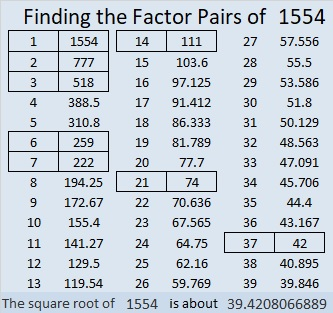# 1554 What Patterns Do You See?

Contents

### Today’s Puzzle:

I like multiples of 111, including 1554. What cool patterns do you notice if a 2-digit number is multiplied by 111 as shown in the graphic below:### A Factor Tree for 1554:

Here’s a factor tree for 1554 that begins with the factor pair 14 × 111:### Factors of 1554:

• 1554 is a composite number.
• Prime factorization: 1554 = 2 × 3 × 7 × 37
• 1554 has no exponents greater than 1 in its prime factorization, so √1554 cannot be simplified.
• The exponents in the prime factorization are 1, 1, 1, and 1. Adding one to each exponent and multiplying we get (1 + 1)(1 + 1)(1 + 1)(1 + 1) = 2 × 2 × 2 × 2 = 16. Therefore 1554 has exactly 16 factors.
• The factors of 1554 are outlined with their factor pair partners in the graphic below.### More about the Number 1554:

1554 is the hypotenuse of a Pythagorean triple:
504-1470-1554, which is (12-35-37) times 42.

This site uses Akismet to reduce spam. Learn how your comment data is processed.